# SBI Clerk Prelims Reasoning Ability Questions 2021 (Day-03)

Dear Aspirants, Our IBPS Guide team is providing new series of Reasoning Questions for SBI Clerk Prelims 2021 so the aspirants can practice it on a daily basis. These questions are framed by our skilled experts after understanding your needs thoroughly. Aspirants can practice these new series questions daily to familiarize with the exact exam pattern and make your preparation effective.

Start Quiz

Designation based puzzle

Direction (1-5): Study the following information carefully and answer the below questions.

Seven persons L, M, N, O, P, Q, and R are working in a restaurant with different grade position such as General Manager (GM), Assistant Manager (AM), Deputy Assistant Manager (DAM), Food Director, Restaurant Manager, Food Runner, and Cashier. Their positions are given in descending order such as General Manager is the highest position & Cashier is the lowest position.

R is a food director. The number of positions between L and P is the same as between R and Q. Two persons are holding the position between N and M. P holds three positions senior to Q. Only one person holds the position between R and N. O neither holds the adjacent position of M nor N.

1) Who among the following person is the Food Runner?

A. the one who holds the immediate junior position of P

B.R

C.O

D. the one who holds the immediate senior position of O

E.None of these

2) Four of the following are alike in a certain way. Which among the following one does not belong to the group?

A. General Manager-L

B. Assistant Manager-N

C. Food director-M

D. Cashier-O

E. Deputy Assistant Manager-P

3) Which of the following statement is/are true?

I). N holds a higher position than L

II). Two persons are holding the position between P and Q

III). O is holding a lower position than Q.

A. Both I and II

B. Only I

C. Both II and III

D. Only II

E. None of these

4) How many persons are holding a higher position than M?

A. Three

B. Two

C. One

D. Five

E. Four

5) If L is related to Assistant Manager and P is related to the Food director in a certain way. Then, in the same way, who among the following is related to Cashier?

A.O

B.Q

C.M

D.N

E. None of the above

Direction sense

Direction (6-8): Study the following information carefully and answer the below questions

Point P is 2m north of Point Q. Point Z is 9m west of Point Q. Point L is 15m east of Point M which is 20m south of Point Z. Point R is 3m south of Point L. Point T is west of point R and South of Point P.

6) How far and what is the direction of Q with respect to T?

A.21m towards the south

B.20m towards the south

C.23m towards the north

D.22m towards the north

E.None of the above

7) What is the shortest distance between Point L and Point Z?

A.21m

B.24m

C.20m

D.23m

E.25m

8) What is the shortest distance between T and R?

A.6m

B.15m

C.7m

D.9m

E.None of the above

Misc

9) If in the given number “2153674562”,1 is subtracted from the odd digit and 2 is added to the even digit of the number, then which of the following digit(s) are repeated more than three times?

A.8

B.4

C.6

D.0

E.2

10) The Position of how many digits in the number “3184697521”will remain unchanged when the digits are arranged in descending order from left to right?

A. Four

B. Three

C. Two

D. One

E. None

Directions (1-5) :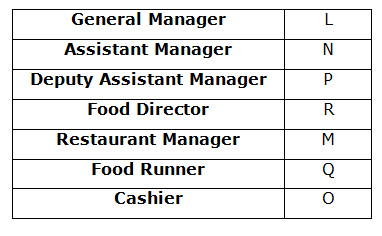R is a food director.

Only one person holds the position between R and N.

From the above condition, there are two possibilities.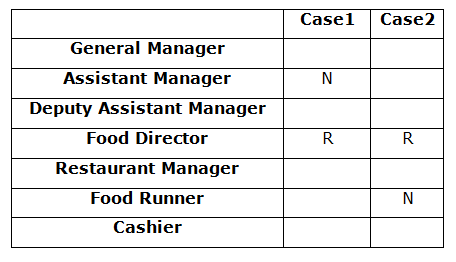Two persons are holding the position between N and M.

O neither holds the adjacent position of M nor N.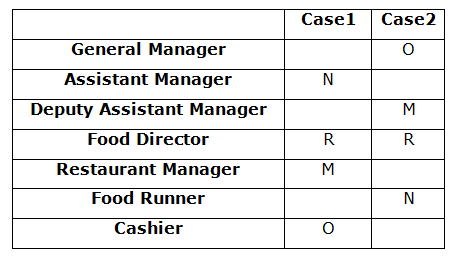P holds three positions senior to Q.

The number of positions between L and P is the same as between R and Q.

From the above condition, case2 gets eliminated. Case1 shows the final arrangement.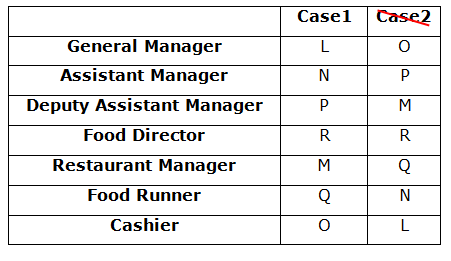Directions (6-8):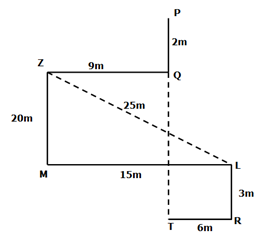202+152=ZL2

√(202+152)=√625=25m

2153674562

4042866484